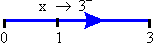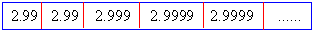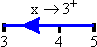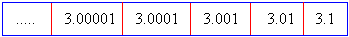Home MonkeyNotes Printable Notes Digital Library Study Guides Study Smart Parents Tips College Planning Test Prep Fun Zone Help / FAQ How to Cite New Title Request

2.3 Limits Of Functions

The concept of limit of a function is a basic concept in calculus. Before studying it, we shall introduce some preliminary concepts.

Meanings of x ® a- , x ® a+ and x ® a

Let a variable x assume values as shown below :It is clear that if x assumes these values, from left to right ( where x < 3 ), the numerical difference between x and 3 gets closer, and closer to 0. In this case, we say that x approaches 3 from left or from below and we write x ® 3-

Similarly let a variable x assume values as shown below:It is clear that if x assumes these values, from right to left ( where x >3 ), the numerical difference between x and 3 gets closer and closer to 0. In this case, we say that x approaches 3 from right or from above and we write x ® 3+.Your browser does not support the IFRAME tag.

However, if x takes values which are very close to 3 and if its values may be greater than or less than 2, then we write x ® 3.

Now in general

• If for any d > 0 and x < a,  x  is  such   that   the interval  0 > a - x > d, then we say that x tends to 'a' from the left, i.e. through values, which are less than 'a' and we write it as

x ® a-

• If for any d > 0 and x > a,   x   is  such  that  the interval 0 > x - a > d, then we say that x tends to 'a' from the right, i.e. through values, which are greater than 'a' and we write it as

x ® a+

• If for any d > 0, x is such that 0 < | x - a | < d, then we say that x ® a.  In  this   case   x  satisfies  the  relation a - d > x > a + d and x ¹ a ( i.e. deleted d-nbd of a ). It is expressed as

x ® a.

• Index

2.1 Modulus
2.2 Inequalities
2.3 Limits Of Functions
2.4 Left Hand And Right Hand Limits
2.5 Theorems On The Algebra Of Limits
2.6 Evaluating Limits
2.7 Limits Of Trigonometric Functions
2.8 The Exponential Limits

Chapter 3